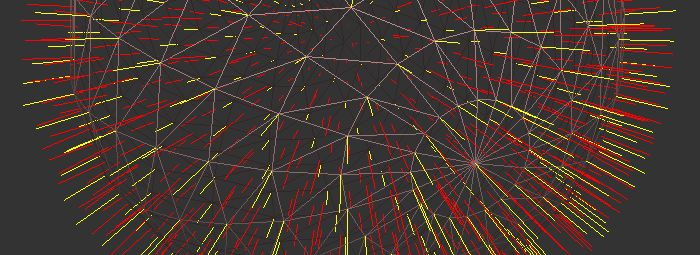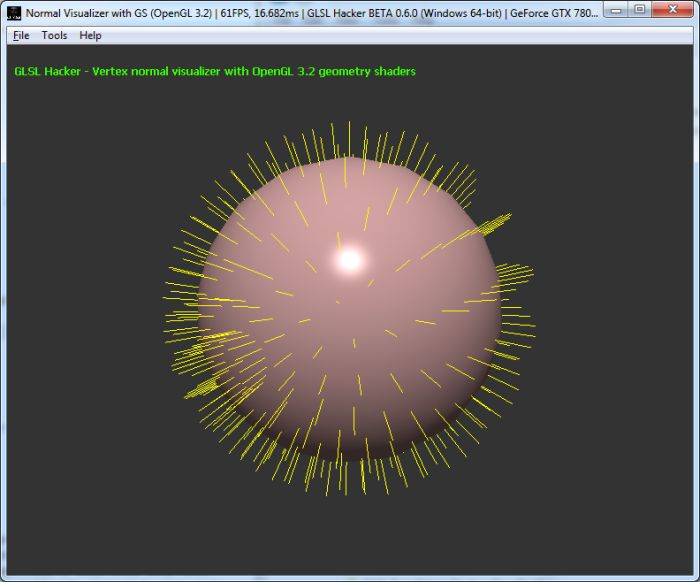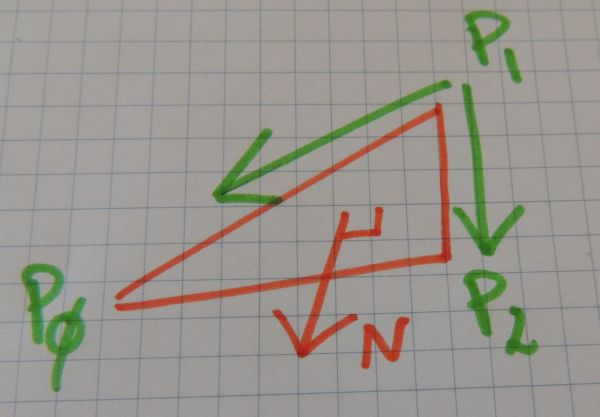Article index:

Hi dear readers, I’m back! Three weeks without new posts… Lot of things to do combined with many sunny days == no post on Geeks3D 😉

Today, we’re going to see a simple but really useful application of geometry shaders: a normal visualizer. I already talked about GLSL geometry shaders HERE and HERE.

A geometry shader allows to create new geometry (a vertex, a line or a polygon) on the fly. We will use this feature to generate lines for visualizing the normals of both vertices and faces of a triangular mesh.

The demos of this article have been coded with GLSL Hacker and you can find all source codes in the Code Sample Pack under the GLSL_Geometry_Shader_Normal_Visualizer/ folder. You can download GLSL Hacker from this page (I recommend to grab the latest DEV version).

As usual, the GLSL programs are not specific to GLSL Hacker. You can use them in any OpenGL/WebGL application with minor changes only (shader inputs).

## 1 – Vertex Normal VisualizerThe generation of the vertex normals is simple. Each normal is a line made up of two vertices. The first vertex is equal to the incoming vertex (which belongs to the current mesh). The second vertex is equal to the first vertex with a displacement along the direction of the vertex normal:

V0 = Pi
V1 = Pi + (normal_length * Ni)

Where i is the vertex index (0 to 2 because the input of the geometry shader is a triangle), Pi and Ni are the position and the normal of the i-th vertex. V0 and V1 are the vertices of the new line.

The vertex normal is part of the geometry shader input vertex. Here is the complete GLSL program (vertex + geometry + fragment) that renders the vertex normals:

This is a simple pass-trough vertex shader. No transformation here, vertices will be transformed for final display in the geometry shader.

```#version 150
in vec4 gxl3d_Position;
in vec4 gxl3d_Normal;

out Vertex
{
vec4 normal;
vec4 color;
} vertex;

void main()
{
gl_Position = gxl3d_Position;
vertex.normal = gxl3d_Normal;
vertex.color =  vec4(1.0, 1.0, 0.0, 1.0);
}
```

The geometry shader does most of the work: it transforms vertices from local space to window space (gxl3d_ModelViewProjectionMatrix) and creates the lines:

```#version 150
layout(triangles) in;

// Three lines will be generated: 6 vertices
layout(line_strip, max_vertices=6) out;

uniform float normal_length;
uniform mat4 gxl3d_ModelViewProjectionMatrix;

in Vertex
{
vec4 normal;
vec4 color;
} vertex[];

out vec4 vertex_color;

void main()
{
int i;
for(i=0; i<gl_in.length(); i++)
{
vec3 P = gl_in[i].gl_Position.xyz;
vec3 N = vertex[i].normal.xyz;

gl_Position = gxl3d_ModelViewProjectionMatrix * vec4(P, 1.0);
vertex_color = vertex[i].color;
EmitVertex();

gl_Position = gxl3d_ModelViewProjectionMatrix * vec4(P + N * normal_length, 1.0);
vertex_color = vertex[i].color;
EmitVertex();

EndPrimitive();
}
}
```

```#version 150
in vec4 vertex_color;
out vec4 Out_Color;
void main()
{
Out_Color = vertex_color;
}
```

## 2 – Face Normal VisualizerThe generation of the vertex normal was simple. Let’s see now how to generate the face normal in the geometry shader. All we need are the thee vertices that define a triangle. We are lucky because those vertices are the inputs of the geometry shader thanks to the following line:

```layout(triangles) in;
```

If P0, P1 and P2 are the positions of the face vertices, the face normal is the result of the following cross product:

```V0 = P0-P1
V1 = P2-P1
N = cross (V1, V0)
```We have now all the theory we need to code the face normal visualizer. Here is the code of the geometry shader alone because compared to the previous GLSL program, only the geometry shader is updated. This geometry shader generates 3 lines for the vertex normals (in yellow) and one line for the face normal (in red): 4 lines or 8 vertices.

```#version 150
layout(triangles) in;
layout(line_strip, max_vertices=8) out;

uniform float normal_length;
uniform mat4 gxl3d_ModelViewProjectionMatrix;

in Vertex
{
vec4 normal;
vec4 color;
} vertex[];

out vec4 vertex_color;

void main()
{
int i;

//------ 3 lines for the 3 vertex normals
//
for(i=0; i<gl_in.length(); i++)
{
vec3 P = gl_in[i].gl_Position.xyz;
vec3 N = vertex[i].normal.xyz;

gl_Position = gxl3d_ModelViewProjectionMatrix * vec4(P, 1.0);
vertex_color = vertex[i].color;
EmitVertex();

gl_Position = gxl3d_ModelViewProjectionMatrix * vec4(P + N * normal_length, 1.0);
vertex_color = vertex[i].color;
EmitVertex();

EndPrimitive();
}

//------ One line for the face normal
//
vec3 P0 = gl_in.gl_Position.xyz;
vec3 P1 = gl_in.gl_Position.xyz;
vec3 P2 = gl_in.gl_Position.xyz;

vec3 V0 = P0 - P1;
vec3 V1 = P2 - P1;

vec3 N = cross(V1, V0);
N = normalize(N);

// Center of the triangle
vec3 P = (P0+P1+P2) / 3.0;

gl_Position = gxl3d_ModelViewProjectionMatrix * vec4(P, 1.0);
vertex_color = vec4(1, 0, 0, 1);
EmitVertex();

gl_Position = gxl3d_ModelViewProjectionMatrix * vec4(P + N * normal_length, 1.0);
vertex_color = vec4(1, 0, 0, 1);
EmitVertex();
EndPrimitive();
}
```

## 3 – References

• OpenGL Superbible, fifth edition, chapter 11

## 3 thoughts on “Exploring GLSL – Normal Visualizer with Geometry Shaders (Shader Library)”

1.Sergey

Good stuff man, I was going to write one of my own by generating the line mesh, but this is so much better. 🙂

2.Mars_999

Welcome back!

thanks good stuff!!

3.Josseline

Hello! I’m new at this programming shaders, so I have a question, I am applying Phong Shading from the vertex shader happened to the fragment shader the vertex normal, if I add the shader geometry (it receives from the vertex normal) and what happened to the fragment is not altered? In case you have primitives that can not be used in the geometry shader not deploy if I add it? Thanks in advance 🙂